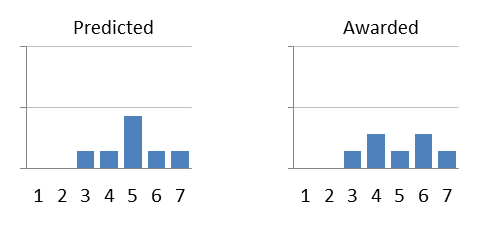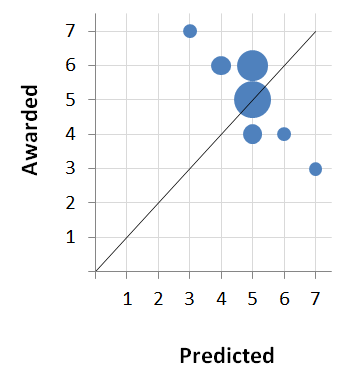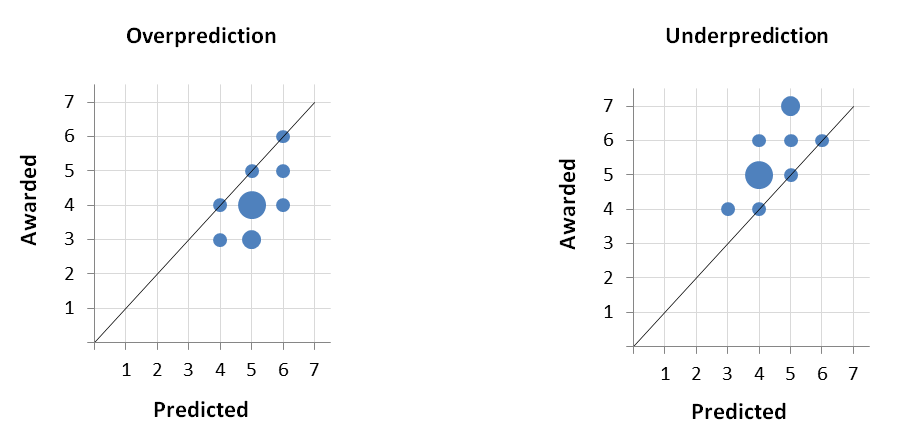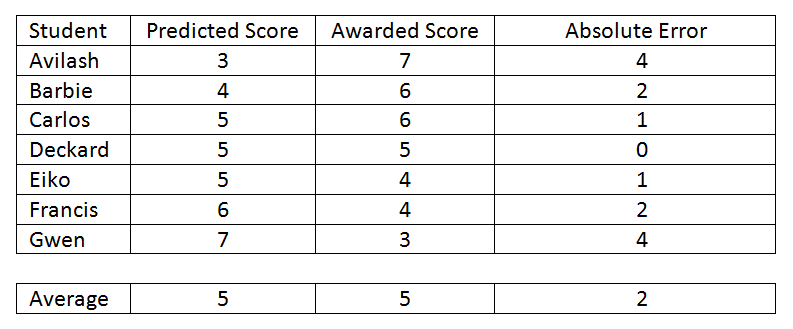# Predicted vs. Awarded Scores

Bubble charts and absolute values are essential for displaying and analyzing predicted vs. awarded scores. This article explains why, and also explains how to interpret them correctly.

Suppose we have a small class of students with the following pairs of predicted and awarded scores.The first thing to notice is that the average predicted score is the same as the average awarded score, and yet only one student had the same predicted and awarded score (Deckard). Thus, comparing the average of the predicted scores to the average of the awarded scores isn't the way to measure the accuracy of the predictions.

Notice also that we cannot simply sum the individual prediction errors represented in the final column. When cases of underprediction (the first three rows) are balanced by cases of overprediction (the last three rows), the sum of the errors may be zero. Again, this is obviously misleading.

Rather than trying to reduce all of the predicted scores to a single number (their average) and doing the same with the awarded scores, perhaps we should compare the two distributions of scores. Like this:The problem here is that we don't know what became of each of the predicted scores. We see that a student was predicted a 3, and we see that a student earned a 3, but were those the same student? Maybe the predicted 3 became the awarded 7? In fact, from the first row of the table, we know that's what happened in Avilash's case.

What we need is a display that keeps the predicted and awarded scores in pairs, so that we can see exactly what happened to each predicted score. Like this:We can see, for instance, that the student who was predicted to earn a 3 was awarded a 7, that just one student was predicted and awarded the same score (5), and that the student who was predicted to earn a 7 was awarded a 3.

What happens if we have more than one student at a given predicted/awarded intersection? We simply increase the size of the bubble. Like this:This shows a large collection of students for whom 5's were predicted and awarded. Several students for whom 5's were predicted and 6's or 4's were awarded, etc.

The diagonal line is included because it represents the locations of accuracy or perfect prediction. Bubbles on the diagonal represent students who had the same predicted and awarded scores.

The diagonal line also allows us to quickly make judgments about trends of overprediction and underprediction. The phrases are slightly counter-intuitive in that bubbles BELOW the diagonal represent cases of overprediction. (The awarded score is lower than the predicted.) Similarly, bubbles ABOVE the diagonal line represent cases of underprediction. (The awarded score is higher than the predicted.)Finally, we can quantify the overall amount of prediction error -- the difference between the predicted and awarded score -- by disregarding the direction of error.

Avilash's awarded score was four points higher than his predicted score, and Gwen's awarded score was four points lower than her predicted score.

Instead of averaging +4 and -4 which leads to zero, we should average the absolute values (4 and 4), like so:We then end up with an "Average Absolute Error" of 2. Meaning, on average, awarded scores were 2 points off of predicted scores. That number no longer includes direction, but that's why we have the bubble chart: to see how the errors were mixed between overprediction, underprediction, and spot-on.

You can see samples of our reports that use bubble charts and average absolute error, here:

Diploma Points - Predicted vs. Awarded Display

Mathematics HL - Predicted vs. Awarded Display

Economics EE - Predicted vs. Awarded Display

TOK - Predicted vs. Awarded Display